# SAT Prep Week 5 Review Mr Crone If

• Slides: 28
Download presentationSAT Prep Week 5 Review Mr. CroneIf (3)(5)(11)y = 11(3)(-15), then y = ?If (3)(5)(11)y = 11(3)(-15), then y = ? 165 y = -495 y = -3If (2)(5)(-7)y = 5(-7)(8), then y = ?If (2)(5)(-7)y = 5(-7)(8), then y = ? -70 y = -280 y=4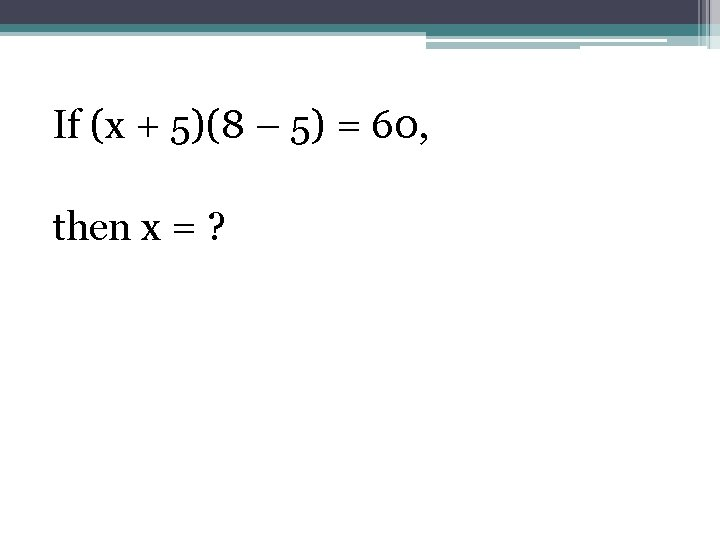If (x + 5)(8 – 5) = 60, then x = ?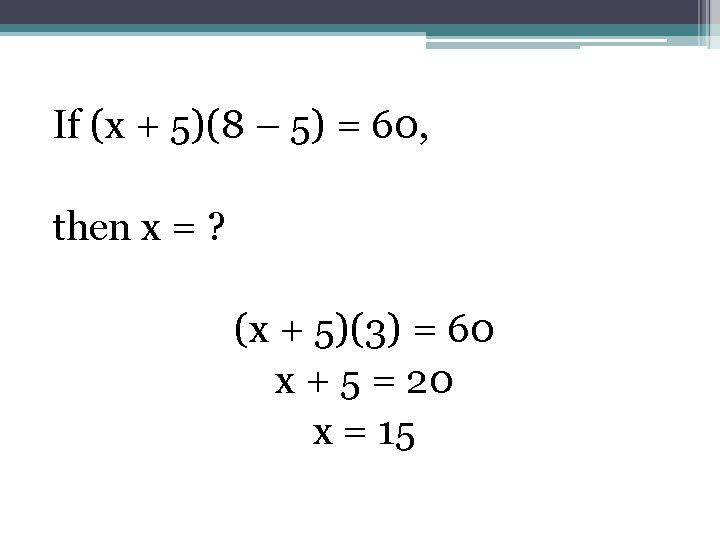If (x + 5)(8 – 5) = 60, then x = ? (x + 5)(3) = 60 x + 5 = 20 x = 15If (r + 6) (9 – 3) = 18, then r = ?If (r + 6) (9 – 3) = 18, then r = ? (r + 6)(6) = 18 (r + 6) = 3 r = -3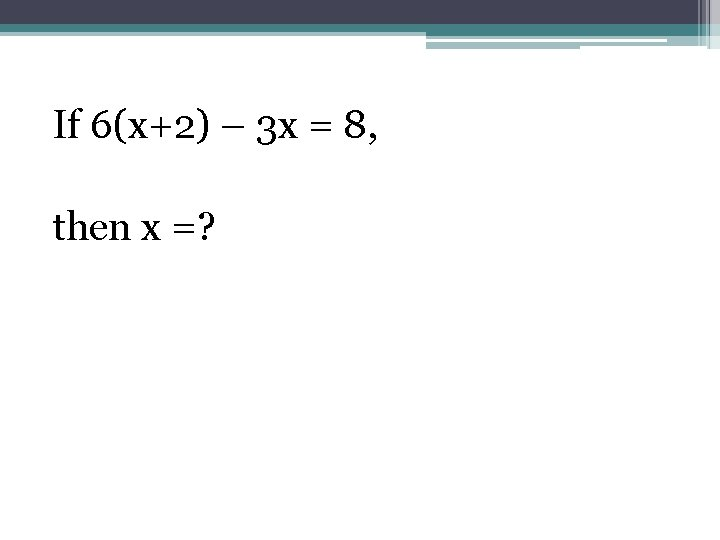If 6(x+2) – 3 x = 8, then x =?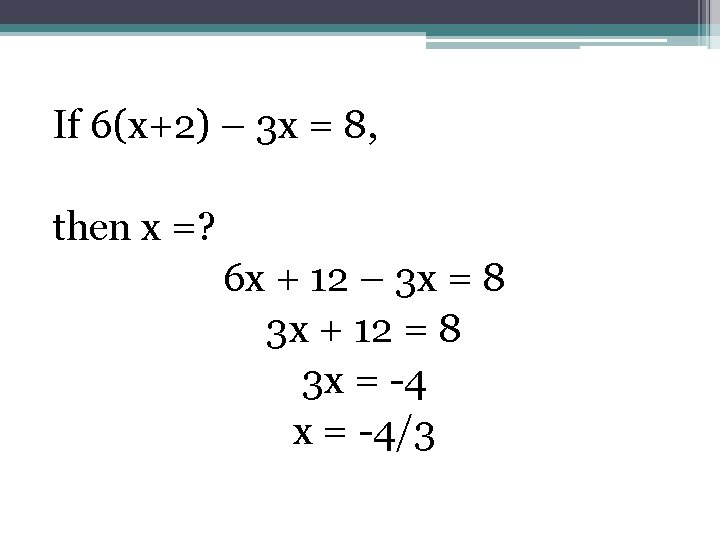If 6(x+2) – 3 x = 8, then x =? 6 x + 12 – 3 x = 8 3 x + 12 = 8 3 x = -4/3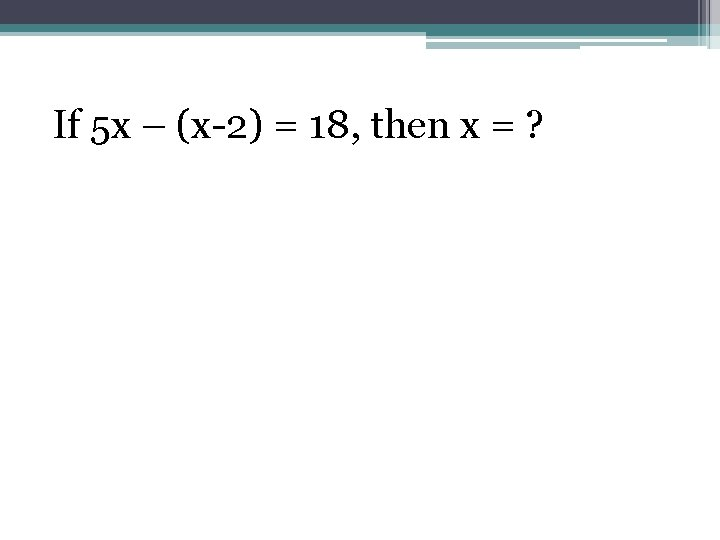If 5 x – (x-2) = 18, then x = ?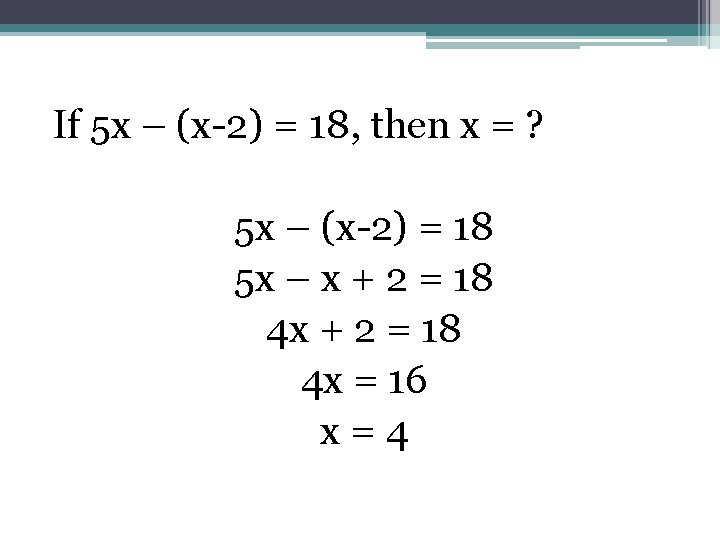If 5 x – (x-2) = 18, then x = ? 5 x – (x-2) = 18 5 x – x + 2 = 18 4 x = 16 x=4If 3 x + 15 = 33, then x + 5 = ?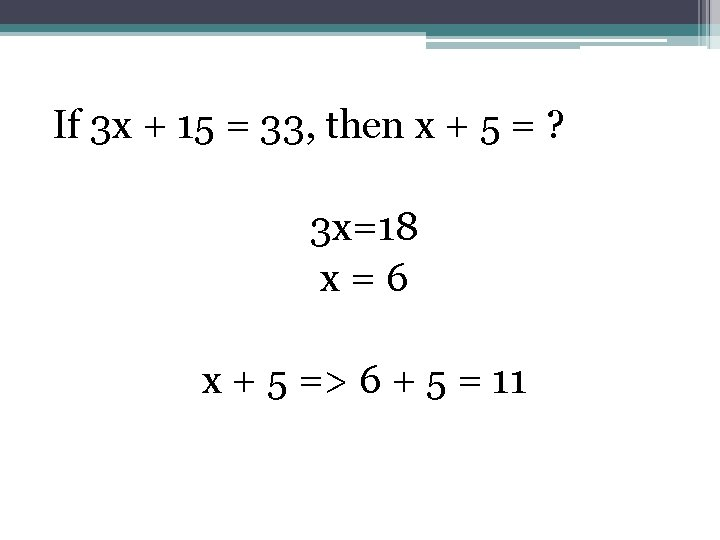If 3 x + 15 = 33, then x + 5 = ? 3 x=18 x=6 x + 5 => 6 + 5 = 11If 4 x – 8 = 6, then 2 x – 4 =?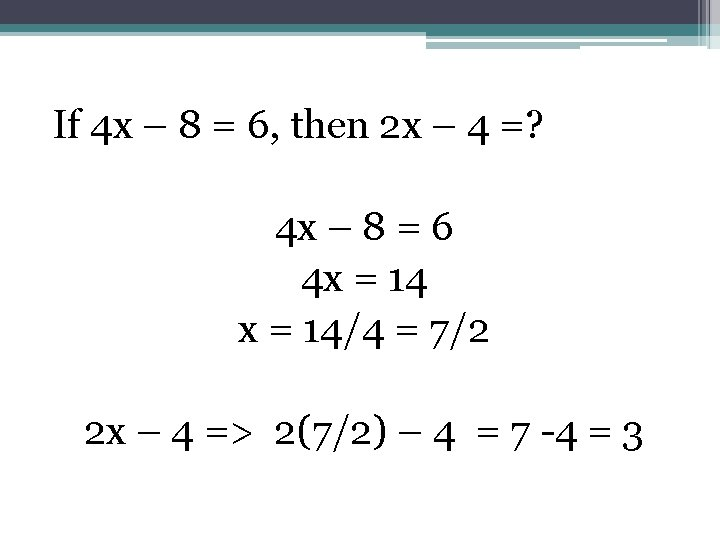If 4 x – 8 = 6, then 2 x – 4 =? 4 x – 8 = 6 4 x = 14/4 = 7/2 2 x – 4 => 2(7/2) – 4 = 7 -4 = 3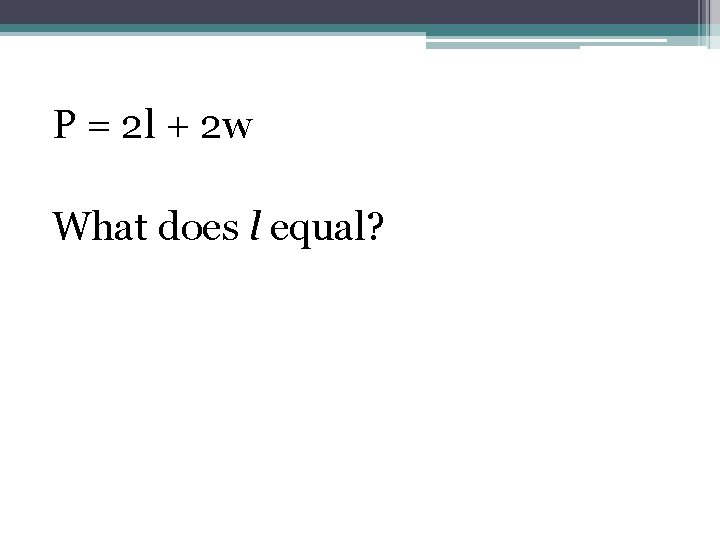P = 2 l + 2 w What does l equal?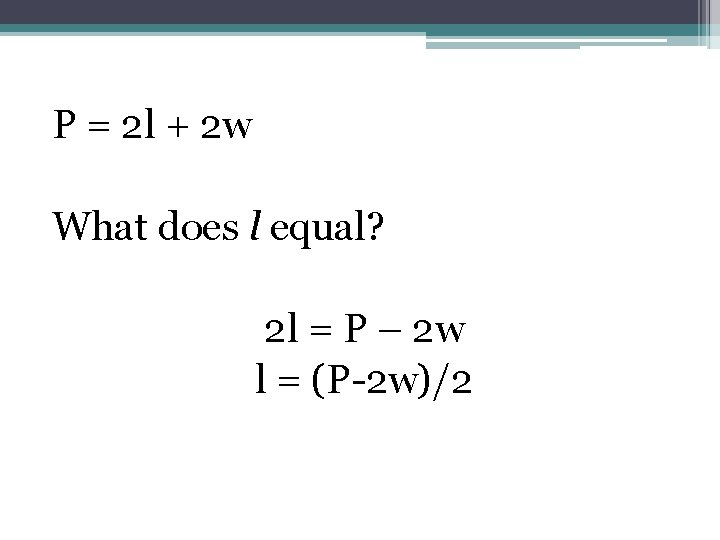P = 2 l + 2 w What does l equal? 2 l = P – 2 w l = (P-2 w)/2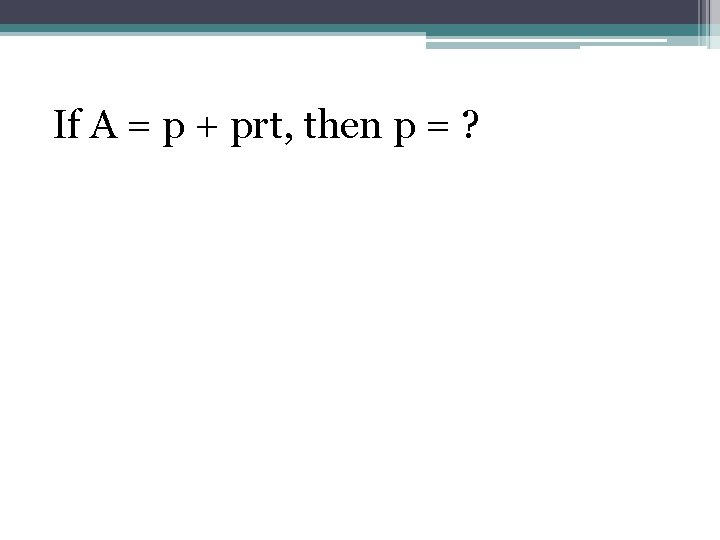If A = p + prt, then p = ?If A = p + prt, then p = ? A = p(1 + rt) A/(1 + rt) = p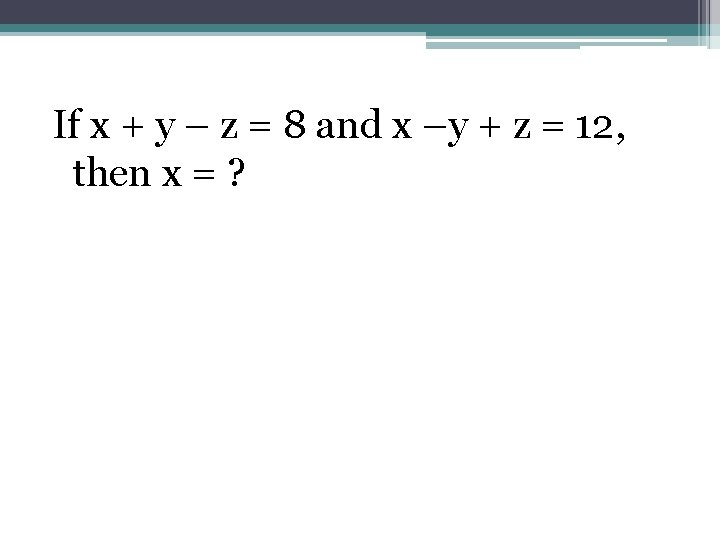If x + y – z = 8 and x –y + z = 12, then x = ?If x + y – z = 8 and x –y + z = 12, then x = ? Solve for y: y = 8 + z – x Substitute: x – (8 + z –x) + z = 12 x – 8 –z + x + z= 12 2 x - 8 = 12 2 x = 20 x = 10If x + 7 = 2 y and y = 2 x -1, what is the value of y?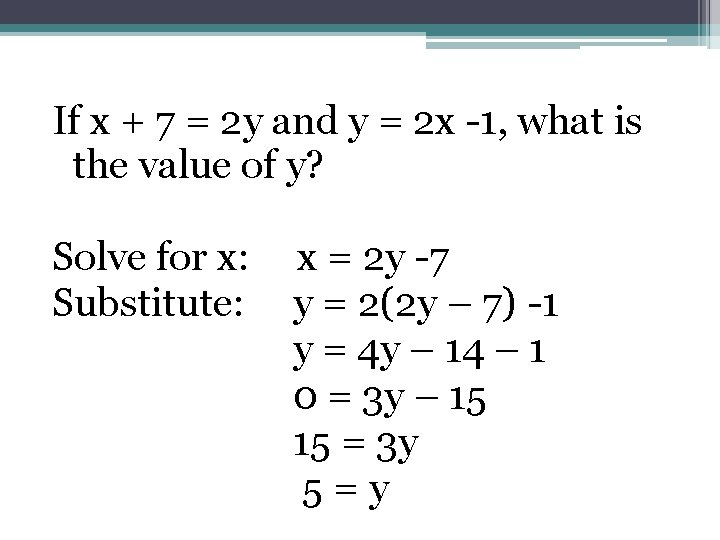If x + 7 = 2 y and y = 2 x -1, what is the value of y? Solve for x: Substitute: x = 2 y -7 y = 2(2 y – 7) -1 y = 4 y – 14 – 1 0 = 3 y – 15 15 = 3 y 5=yIf 2 x + y = 6, and x -6 = y, what is the value of x?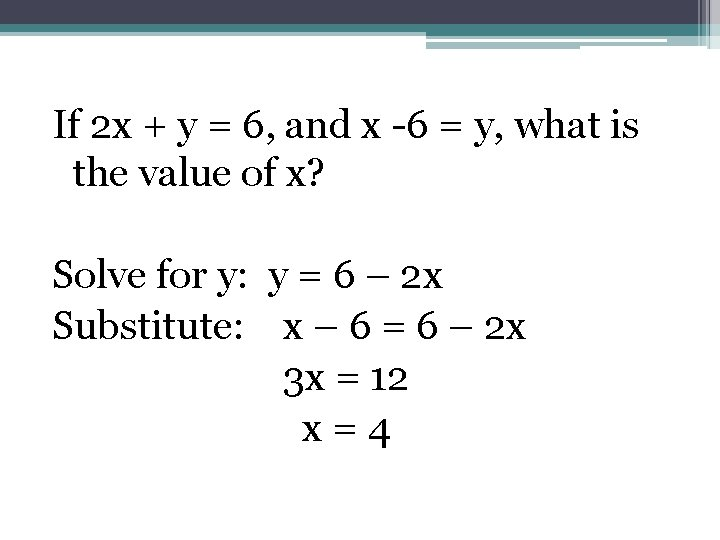If 2 x + y = 6, and x -6 = y, what is the value of x? Solve for y: y = 6 – 2 x Substitute: x – 6 = 6 – 2 x 3 x = 12 x=4If 2 x – y = 0 and x – 2 y = 3, then 6 x – 6 y = ? Solve for y: y = 2 x Substitute: x – 2(2 x) = 3 x – 4 x = 3 x = -1 and y = -2 Solve: 6(-1) – 6(-2) = -6 + 12 = 6